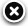# NavList:

## A Community Devoted to the Preservation and Practice of Celestial Navigation and Other Methods of Traditional Wayfinding

Message:αβγ
Message:abc
 Add Images & Files Name or NavList Code: Email:
Re: Distant Horizon
From: Frank Reed
Date: 2023 May 18, 19:17 -0700

Hello Herman,

"Is there a table or formula to calculate the "reduction factor" for different heights above sea level. I am interested because when I am in Germany I take sights between 450 and 800 mtr. "

Yes. For low altitudes above sea level, below let's say 1 or 2 km, the scaling factor is approximated reasonably well by taking the observer height, h, in km and dividing by a standard scale height, H, of 9.5 km. At higher altitudes, use the "exponential function", ex, on that same ratio.

Example: suppose my height is 475m above sea level. Then h/H is 0.05. This means that refraction at that altitude is reduced by 5%. If the tables say refraction is, let's say, 4.0', then at that highland location you would use 3.8'. You can see that this would mostly be an irrelevant correction except for celestial objects below 5 or 10° in altitude above the horizon.

Higher altitude example: Suppose I am 3800m above sea level. The ratio h/H is now 0.4. If we use the lower altitude approximation, we would guess that refraction is reduced by 40%. But if you punch this up on your calculator [a scientific calculator almost certainly has ex or equivalently exp(x) as the inverse of the natural logarithm, ln(x)], then you'll find that exp(-0.4) is 0.67. If the tables for sea level refraction list 4.0' then you want two-thirds of that, or 2.7'.

Very high altitude example: Suppose I am 9500m above sea level in an aircraft. The lower altitude approximation would say that refraction is zero here, as if the atmosphere vanishes at 9.5km height. But the proper exponential factor is exp(-1), which is 1/2.718, which is 0.37 instead of zero. Refraction is reduced by that factor.

There are a variety of other formulae for the altitude correction factor with various assumptions to them. The ones I'm describing here are the ones that I use regularly, and they are close enough for nearly any purpose.

"Or gives the local air pressure on that height already a sufficient correction?"

Yes, but it's an either/or. If you have a barometer that has been calibrated at sea level, and if it has been transported to your altitude above sea level, then you can use its pressure reading directly. Of course, most of us get pressure from other sources, and those sources are usually calibrated to show air pressure "as if" the observing location is at sea level. So if you have a "normal" reported pressure (like from a weather report), then you should apply a correction for altitude, as above. If you have no reported pressure and you want a "mean" correction, then correct the tables, as above. If you have actual local air pressure, which is rare, then you would use that local pressure without a correction for altitude.

Frank ReedBrowse Files

Drop Files### NavList### What is NavList?### Get a NavList ID Code

 Name: (please, no nicknames or handles) Email:
 Do you want to receive all group messages by email? Yes No
A NavList ID Code guarantees your identity in NavList posts and allows faster posting of messages.### Retrieve a NavList ID Code

Enter the email address associated with your NavList messages. Your NavList code will be emailed to you immediately.
 Email:### Email Settings

 NavList ID Code:### Custom Index

 Subject: Author: Start date: (yyyymm dd) End date: (yyyymm dd)